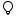Use our forex spread calculator to find out the spread cost of your leverage forex trading pair. It can be used for any forex pair, simply follow the step-by-step guide below.

How to use the forex spread calculator:

2. Add the Bid Price. (e.g. 1.0095 or 1.5233)
3. The forex spread is calculated automatically.Information: A low spread cost in the Forex market is between 0.06% – 0.03%. A high spread cost in the Forex market is considered anything above 0.10%.

## What is a forex spread calculator?

A forex spread calculator is a tool designed to help traders figure out the difference between the bid and the ask price of a currency pair in the forex market.

This difference, known as the spread, is very important in forex trading, influencing the potential profit and loss from trades.

### How the forex spread calculator works

Using the forex spread cost calculator is straightforward:

• Ask Price: Input the current ask price of the currency pair you’re interested in.
• Bid Price: Provide the current bid price for the currency pair.
• Calculate: The calculator automatically determines the spread percentage, giving you a clear idea of the cost to enter a trade.

### What is the formula for calculating forex spread?

The formula to calculate the forex spread is simple:

• Ask Price: This is the minimum price that a seller is willing to accept for a currency pair.
• Bid Price: This is the maximum price a buyer is ready to pay for the currency pair.

### How do you calculate the spread in forex?

To calculate the Forex spread, perform the following steps:

• Input the Prices: Insert these values into the appropriate fields on the calculator.
• View the Result: The calculator automatically showcases the spread in percentage terms, helping you to understand the prevailing market conditions.

### Example of calculating forex spread

Let’s say the current ask price for a currency pair is 1.1000, and the bid price is 1.0990.

Using our formula, we find the spread to be:

Forex Spread = ((1.1000 – 1.0990) / 1.1000) x 100% ≈ 0.091%

This reveals a relatively low spread, which is generally seen as good, especially when working with an optimal forex lot size and leverage.

### Why you should calculate forex spread

Calculating the Forex spread is vital for several reasons:

• Cost-Efficiency: Keeping the spread low can save you money in the long run.